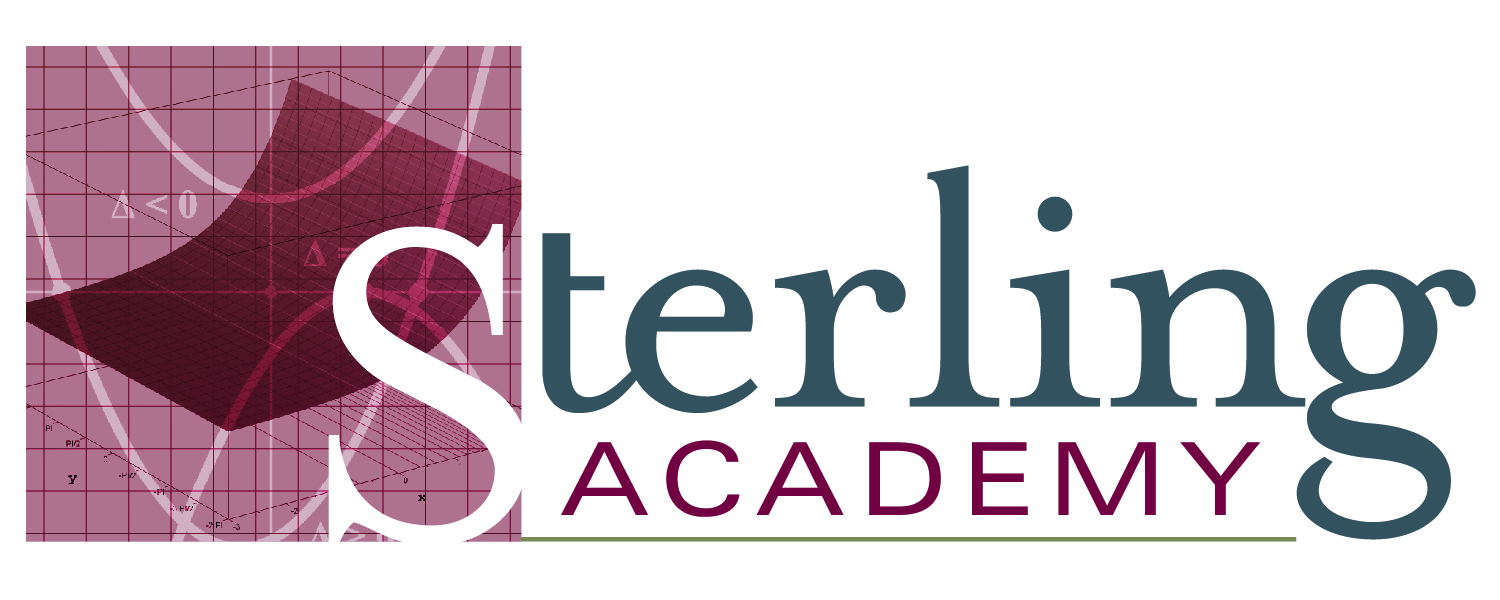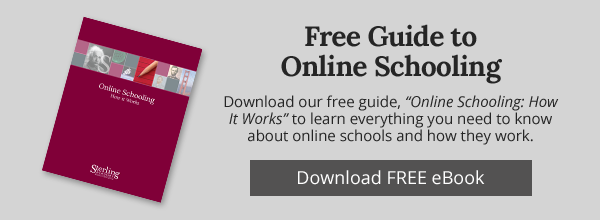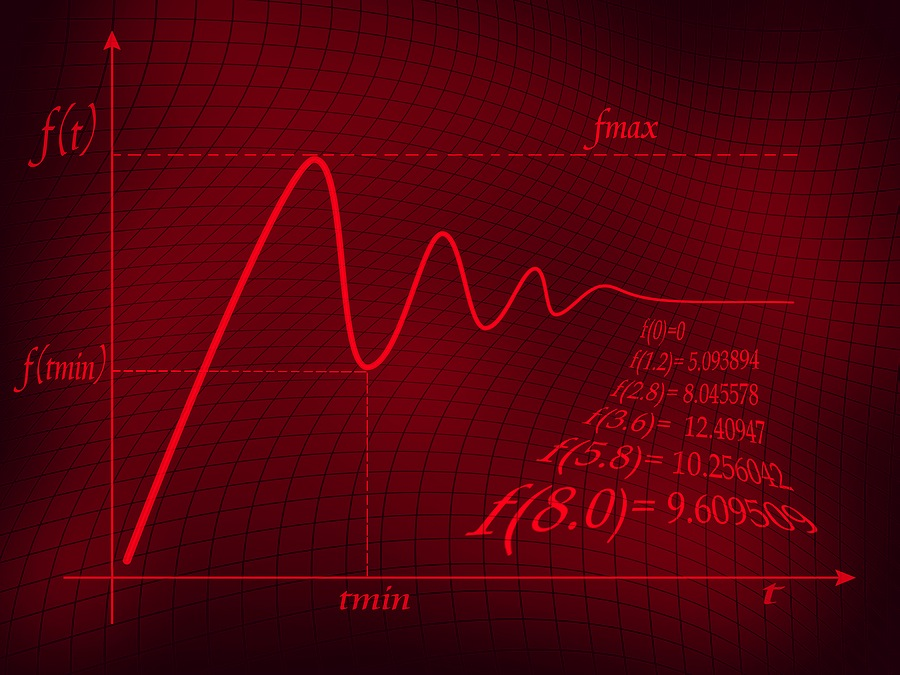<img height="1" width="1" alt="" style="display:none" src="https://www.facebook.com/tr?id=821992654522188&amp;ev=PixelInitialized">

# Online High School Math Curriculum

Accredited Online High School Math Curriculum.Students who study math online at Sterling Academy can learn in a friendly, encouraging environment. Many tools are provided to tailor learning to the individual student to make each learning experience customized and individualized.  Open communication with feedback keeps students on top of their learning with clear knowledge of their progress.  Having self-directed math courses allows students to take ownership of their education while they develop pride and confidence.

Here is a short demonstration video from our Algebra 1 course.

## SAMPLE High School Math Course Descriptions

Algebra 1

This course provides an in depth coverage of  writing, solving, and graphing a variety of equations and inequalities, linear systems, functions, properties of real numbers, and numeric and algebraic expressions, including laws of exponents, radicals and rational expressions.

Lab activities allow students to explore algebraic principles.  For example, in the lesson on Solving Equations, students interactively solve equations using the distributive property and then graph them.

Geometry

This course consists of reasoning, proofs, basic constructions, triangle relationships, quadrilaterals, and the concepts of surface area, volume, circles, and transformations.

Complementary labs strengthen the lessons in this course.  For example, in the lesson on Properties of Triangles, students interactively classify triangles based on their sides and angles.

Algebra 2

Students master advanced algebraic concepts, including trigonometry, statistical analysis, permutations, and sequences and series.

Students build on their learning through lab exercises.  For example, in the section on Operations with Functions, students are able to create and graph functions interactively.

Honors Pre-Calculus

Exploring the relationship between advanced algebra topics and trigonometry, Pre-calculus is an informative introduction to calculus that challenges students to discover the nature of graphs, nonlinear systems, and polynomial and rational functions. With an emphasis on mathematical reasoning and argument, this advanced course scaffolds rigorous content with clear instruction and an array of scaffolds for learning, providing students with a deep understanding of topics such as matrices, functions, graphing, logarithms, vectors, and conics. The course concludes with a brief introduction to calculus that exposes students to limits, continuity, derivatives, and the Fundamental Theorem of Calculus.